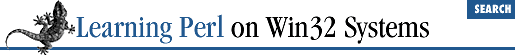home | O'Reilly's CD bookshelfs | FreeBSD | Linux | Cisco | Cisco Exam

### 9.6 &&, ||, and ?: as Control Structures

These look like punctuation characters, or parts of expressions. Can they really be considered control structures? Well, in Perl-think, almost anything is possible, so let's see what we're talking about here.

Often, you run across "if this, then that." We've previously seen these two forms:

```if (```

this
```
) { ```

that
```
; } # one way
```

that
```
if ```

this
```
;       # another way```

Here's a third (and believe it or not, there are still others):

``````

this
```

&& ```

that
```
;```

Why does this statement work? Isn't that the logical-and operator? Check out what happens when ``` this ``` takes on each value of true or false:

• If ``` this ``` is true, then the value of the entire expression is still not known, because it depends on the value of ``` that ``` . So ``` that ``` has to be evaluated.

• If ``` this ``` is false, there's no point in looking at ``` that ``` , because the value of the whole expression has to be false. Because you don't have to evaluate ``` that ``` , we might as well skip it.

And in fact, Perl does just that. Perl evaluates ``` that ``` only when ``` this ``` is true, making the form equivalent to the previous two examples.

Likewise, the logical ``` or``` works like the ``` unless``` statement (or ``` unless``` modifier). So, you can replace:

```unless (```

this
```
) { ```

that
```
; }```

with

``````

this
```

|| ```

that
```
;```

Finally, the C-like ternary operator:

```

```

exp1
```
? ```

exp2
```
: ```

exp3
```
;```

evaluates to ``` exp2 ``` if ``` exp1 ``` is true, and to ``` exp3 ``` in all other cases. You might have used:

```if (```

exp1
```
) { ```

exp2
```
; } else { ```

exp3
```
; }```

but you could have eliminated all of that punctuation. For example, you could write:

`(\$a < 10) ? (\$b = \$a) : (\$a = \$b);`

Which one should you use? Your choice depends on your mood, sometimes, or on how big each of the expression parts are, or on whether you need to parenthesize the expressions because of precedence conflicts. Look at other people's programs, and see what they do. You'll probably see a little of each. Larry suggests that you put the most important part of the expression first, so that it stands out.9.5 Expression Modifiers9.7 Exercises